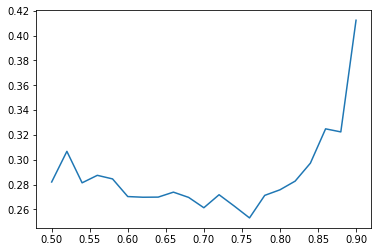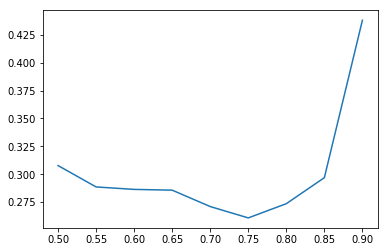# Dropout finder?

I am wondering what people are using to pick a dropout rate.

I am instead offering a modest approach that avoids training a full model for each possible dropout rate.

We are relying on the fact that running with precomputed activations is much faster and we can therefore run a lot of epochs with little cost.

I am suggesting to just loop through the possible dropout rates and run e.g. 10 epochs:

``````num_epochs=10

lr=1e-1

dropout_rate=[]
min_val_loss=[]
learn.save('temp')
for ps in np.arange(0.5,0.91,0.02):

dropout_rate.append(ps)
learn = ConvLearner.pretrained(arch, data, precompute=True,ps=ps)
_, ep_vals = learn.fit(lr,num_epochs,get_ep_vals=True);
min_val_loss.append(min(l for l in list(ep_vals.values())))

plt.plot(dropout_rate, min_val_loss)
``````

Code could be improved, for example I wonder how to hide the displayed results as the script is running.

This is an example of what I get on the dog breeds data, with resnext101_64. We are plotting best training loss vs. dropout rate.Suggested dropout rate would be 0.77.

I suppose this would only work for the connected layers that we are adding to an existing architecture (so we can use precomputed activations).

Some things to try:

• use SGDR to go a bit deeper with each dropout rate
• try a different dropout rate for each FC layer (note: by default we have 0.25 and 0.5)

Looking forward to all your suggestions!

7 Likes

Here is a smoother chart (min(val loss) vs dropout rate) with 0.05 intervals in dropout rate.Really interesting idea. I’m going to try this out on my own model. It’s using structured data and only 2 fully connected layers. I’ll report back results when i get it working.

Thanks for the interest. Let me know if you get any results or insights.

I’ve also experimented with changing ps in the midst of training, but didn’t get any positive results.

For the dog breeds with resnext101_64, picking the right dropout rate, rather than just 0.5, seemed to make a significant difference in the final result.

Has anyone tried using a dynamic dropout rate? I’m thinking of a system similar to the learning rate finder. You would start with a dropout-keep of 1 (i.e. no dropout) and gradually go to 0. The loss vs dropout plot should also have a U shape. It could potentially help find the best dropout.

Hey that’s a good idea. Might also want to try going the other way (high drop out to no drop out) and see what works best. There is a risk of initially overfitting if you start with no drop out.

Nice work here! Just to make sure I understand – will the precomputed activations work for any trained model weights? In my limited usage, I’ve found increasing dropout to be helpful only after I’ve started overfitting.

Over-fitting should not be an issue if you keep the number of training steps low. With learning rate finder I increase the learning rate after each gradient step and do less than 10k steps (~ 1 epoch of my dataset).
Going from high dropout to no dropout may be less interpretable because the plot would just go down.

The dog breeds data is a bit special as the upper layers don’t need to be retrained. I’ve found that I could overfit even in the precompute=true phase.